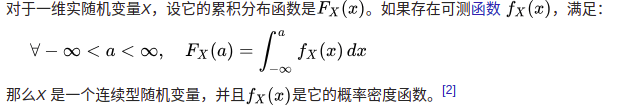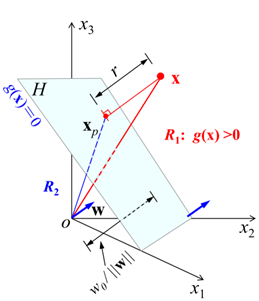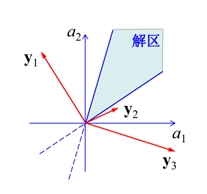• 贝叶斯公式条件概率推出，我们假设要做一个分类任务，给出数据A求它的标签B，这就是公式左边。直接求解比较困难，所以贝叶斯公式可以把它转化成P(A|B)，即在标签B条件下是数据A的概率。 ...
1.条件概率

条件概率反应的是在给定A的条件下B的概率

由条件概率可得

由此还可以推出全概率公式，在全概率公式里，P(A)是所有P(AB_i)的求和，对应概率图表中A的偏概率

2.贝叶斯公式

贝叶斯公式由条件概率推出，我们假设要做一个分类任务，给出数据A求它的标签B，这就是公式左边。直接求解比较困难，所以贝叶斯公式可以把它转化成P(A|B)，即在标签B条件下是数据A的概率。

贝叶斯定理形式为，它让我们能够通过后验概率p(w|D)，在观测到D之后估计w的不确定性。

3. 先验概率 后验概率

现在来举个例子说明，我们考虑这样一个文本分类的问题，x是文章的向量，y是文章的类别，在给出训练集的情况下，显然P(y|x)是我们要求的，这个不能直接求得。但是p(x),p(y),p(x|y)都是可以在训练集上统计出的。我们写出这个问题的贝叶斯公式，其中

P(y)是先验概率，先验概率顾名思义，是人们的先天经验，是在没有给出数据集前对结果的估计

P(y|x)是后验概率，也就是我们要求的概率，它的含义是当我们给出数据之后发现先验假设存在偏差，是我们观测到x之后的概率。

关于后验概率，也许上面的例子还不太直观，我们考虑有两个盒子,一个红色的,一个蓝色的,红盒子中有2个苹果和6个橘子,蓝盒子中有3个苹果和1个橘子。现在假定我们随机选择一个盒子,从这个盒子中我们随机选择一个水果,观察一下选择了哪种水果,然后放回盒子中。假设我们重复这个过程很多次。假设我们在40%的时间中选择红盒子,在60%的时间中选择蓝盒子,并且我们选择盒子中的水果时是等可能选择的。当我们拿了一个水果后，要判断它是从哪个盒子里拿的

在这个例子中，我们要求的是P(b|f)，即在给定fruit下预测是从哪个盒子里拿的。先验概率就是P(b)，因为我们知道选蓝盒子的概率是0.6，所以在没有选水果前我们就会预先猜测更有可能从蓝盒子里取。

但是现在我拿了一个水果发现是橘子，选蓝盒子就不是0.6了，因为我们知道红盒子里橘子更多，所以感觉应该是红盒子更有可能，因为蓝盒子只有1个橘子。先验概率因为我们的观测而产生了变化，这个就是后验概率。现在我们再算一下红蓝盒子的概率：

4.似然函数

L(θ|x)=f(x|θ)

似然函数的意思是当给定一个样本x后，我们去猜想它在分布的不同参数下出现的概率。统计学认为数据是在一个给定的分布下生成的，而我们要找的就是分布的参数。f(x|θ)表示的就是在给定参数theta的情况下，x出现的可能性多大。L(θ|x)表示的是在给定样本x的时候，哪个参数theta使得x出现的可能性多大。

在上面的例子中，似然函数就是P(x|y)，我们求最大似然，就是求在给定标签y时，看生成数据x的可能，然后找可能最大的那个y，注意似然函数并不是归一化的。

5.概率分布与概率密度

当我们研究随机变量的时候，我们关注的将不仅是取哪些值，还要看它取到各种值的概率。在上面的例子中，我们能取到的值都是离散的，如果我们要考虑连续的值呢，例如女朋友约我10点见面，我要算什么时候去最能讨女友欢心，时间是一个连续的值，这时就要引入概率分布和概率密度。

首先，先有概率分布后有概率密度，可以看到，概率密度是概率分布的导数。对于连续型随机变量，我们考虑的更像是一根铁棍各处的密度，密度大的地方当我们积分的时候概率就大。参考

https://blog.csdn.net/yangang908/article/details/62215209   先验后验

https://www.jianshu.com/p/b570b1ba92bb   概率分布 概率密度

https://www.zhihu.com/question/54082000  似然函数


展开全文• 文章目录前言一、文章重点及流程梳理二、概率论基础知识三、参数估计1....1、介绍这部分所设计的概率论知识，包括条件概率、全概率、事件独立性、贝叶斯公式。 2、 3、 二、概率论基础知识 1.条件概
文章目录前言一、文章重点及流程梳理二、概率论基础知识三、参数估计1.极大似然估计（Maximum Likelihood Estimation）2.贝叶斯估计

前言
写作参考概率论书籍、西瓜书、李航《统计学习方法》及其他资料，若有不足请大家不吝赐教！

一、文章重点及流程梳理
本文目的在于：
1、阐述MLE参数估计的思想，并计算参数在正态分布下的估计量
2、阐述贝叶斯估计的思想，并介绍贝叶斯估计与MAP的不同点
流程梳理：
1、介绍这部分所涉及的概率论知识，包括条件概率、全概率、事件独立性、贝叶斯公式。
2、介绍MLE并求解参数在正态分布下的估计量，并进行比较。
3、介绍贝叶斯估计及MAP的思想。
二、概率论基础知识
1.条件概率
$P\left( B|A \right) \,\,=\,\,\frac{P\left( AB \right)}{P\left( A \right)}\,\, \left( 1 \right)$
通过下图对上式进行描述：
图中有两集合A、B，黄色部分为A、B的交集部分。则P(B|A)表示在A发生的情况下，B发生的概率，可以通过交集部分发生概率占A所发生概率的比值表示。同理，若要求P(A|B)只需要换成交集部分发生概率占B所发生概率的比值。2.事件独立性
定义：在一次试验中，一事件发生与否与另一事件是否发生无关。满足下式：
$P\left( AB \right) \,\,=\,\,P\left( A \right) P\left( B \right) \,\, \left( 2 \right)$
则称A、B相互独立。
PS:独立同分布指的是随机变量服从同一分布且相互独立。
3.全概率公式
$P\left( A \right) \,\,=\,\,\sum_{i\,\,=\,\,1}^n{P\left( B_i \right)}P\left( A|B_i \right) \,\, \left( 3 \right)$
公式解读：若A事件的发生可由多项B事件引起，那么这时候A发生的概率等于B事件发生的概率乘以B事件发生条件下A发生概率之和。
举个栗子：假设A是今天感到快乐的概率，可以通过吃东西B1，或者是买了新衣服B2，或者是出了考试成绩B3，或者是有人和自己告白B4。那么，所有的B事件发生，需要一定的概率；在B事件发生得概率下，开心和不开心都存在可能，而我们只取B事件下开心的概率，这时候A要发生的概率，就是所有B事件发生概率*B事件下A发生得概率的和。
4.贝叶斯公式（逆概公式）
贝叶斯公式的初始形式：
$P\left( B|A \right) \,\,=\,\,\frac{P\left( A|B \right) P\left( B \right)}{P\left( A \right)}$
其中，P(A|B)称为似然（likelihood），P(B)称为先验（prior），P(A)称为事实，P(B|A)称为后验（posterior）。
后验P(B|A)求的是在A发生条件下，B发生得概率；似然P(A|B)求的是，若A发生则B作为影响因子出现的概率。

通过式（1）和式（2），可得到如下贝叶斯公式的变形：
$P\left( B_j|A \right) \,\,=\,\,P\left( B_j \right) \,\, ·\,\, \frac{P\left( A|B_j \right)}{\sum_{i\,\,=\,\,1}^n{P\left( B_i \right)}\,\,P\left( A|B_i \right)}\,\, \left( 4 \right)$
通过下图对上式进行理解：整个圆划分为三个部分A、B、C，黄色部分为M集合，若此时求M发生A中的概率，则
$P\left( A|M \right) \,\,=\,\,\frac{P\left( A\cap M \right)}{P\left( M \right)}\,\,$
$=\,\,\frac{P\left( M|A \right) P\left( A \right)}{P\left( M|A \right) PA+P\left( M|B \right) P\left( B \right) +P\left( M|C \right) P\left( C \right)}$
通过例子可知，后验概率目的在于，已知M发生后，想知道由A引发M事件的概率，即为：知道结果后反推原因。
三、参数估计
1.极大似然估计（Maximum Likelihood Estimation）
1、MLE思想
频率派角度：认为参数是固有的，但是可能由于一些外界的噪声干扰，使数据看起来不是完全由参数决定。但只要在这个数据给定的情况下，找到一个概率最大的参数就可以了。即，模型已定，参数未定。
$P\left( x|\theta \right)$
2、MLE表示形式
当存在多个样本时，需要多个似然相乘，此时样本间独立同分布，即：
$P\left( D_c|\theta _c \right) \,\,=\,\,\prod_{x\in D_c}{P\left( x|\theta _c \right)}$
对式子取对数得到：
$LL\left( \theta _c \right) \,\,=\,\,\sum_{x\in D_c}{\log P\left( x|\theta _c \right)}$
则可以得到极大似然估计的表达式：
$MLE\,\,=\,\,arg\,\,\underset{\theta _c}{\max}P\left( D_c|\theta _c \right) \,\,=\,\,arg\,\,\underset{\theta _c}{\max}\,\,LL\left( \theta _c \right)$试图在θ的所有取值中，找到一个使式子最大化的θ。
3、求解极值
假设参数θ满足正态分布，即 θ =（μ，∑），在一维情况下，θ =（μ，∑^2）
则有：$MLE\,\,=\,\,arg\,\,\underset{\theta _c}{\max}\,\,\sum_{i\,\,=\,\,1}^N{P\left( x_i|\theta \right) \,\,=\,\,arg\,\, \underset{\theta _c}{\max}\,\,\sum_{i\,\,=\,\,1}^N{\frac{1}{\sqrt{2\pi}\sigma}e^{-\frac{\left( x_i-\mu \right) ^2}{2\sigma ^2}}}}$
此时，问题转换成了求参数θ的MLE。

step 1：求μ的极值
$\frac{\partial \mu}{\partial \theta}\,\,=\,\,\left( -\sum_{i\,\,=\,\,1}^N{\,\,·\,\,\frac{1}{2}\log 2\pi \,\,-\,\,\sum_{i\,\,=\,\,1}^N{\log \sigma}\,\,-\,\,\sum_{i\,\,=\,\,1}^N{\frac{\left( x_i-\mu \right) ^2}{2\sigma ^2}}} \right) _{\mu}^{\prime} \\ =\,\,\sum_{i=\,\,1}^N{\frac{2\left( x_i-\mu \right)}{2\sigma ^2}}\,\,$

令上式取0，得：
$\sum_{i\,\,=\,\,1}^N{x_i\,\,=\,\,\sum_{i\,\,=\,\,1}^N{\mu \,\, \Longrightarrow \,\, \mu _{MLE}\,\,=\,\,\frac{1}{N}\sum_{i\,\,=\,\,1}^N{x_i}}}$

step 2：求δ的极值
$\frac{\partial \sigma}{\partial \theta}\,\,=\,\,\left( -\sum_{i\,\,=\,\,1}^N{\,\,·\,\,\frac{1}{2}\log 2\pi \,\,-\,\,\sum_{i\,\,=\,\,1}^N{\log \sigma}\,\,-\,\,\sum_{i\,\,=\,\,1}^N{\frac{\left( x_i-\mu \right) ^2}{2\sigma ^2}}} \right) _{\sigma}^{\prime} \\ =\,\,-\sum_{i\,\,=\,\,1}^N{\frac{1}{\sigma}}\,\,+\,\,\sum_{i\,\,=\,\,1}^N{\frac{\left( x_i-\mu \right) ^2}{\sigma ^3}}\,\,$

上式取0，得：
$\sum_{i\,\,=\,\,1}^N{\frac{1}{\sigma}\,\,=\,\,\sum_{i\,\,=\,\,1}^N{\frac{\left( x_i-\mu \right) ^2}{\sigma ^3}\,\, \Longrightarrow \,\, \sigma ^2_{MLE}\,\,=\,\,\frac{1}{N}\sum_{i\,\,=\,\,1}^N{\left( x_i-\mu _{MLE} \right) ^2}}}\,\,$
4、MLE估计结果
判断在参数为正态分布的情况下，所得到的估计与实际是否一致。
$E\left( \mu _{MLE} \right) \,\,=\,\, \frac{1}{N}\sum_{i\,\,=\,\,1}^N{E\left( x_i \right)}\,\,=\,\,\frac{1}{N}\sum_{i\,\,=\,\,1}^N{\,\,·\mu}\,\,=\,\,\mu$
对所得的均值求期望，得到的结果为，正态分布下的均值为样本均值，与正太分布下的均值相同，为无偏估计。对所得的方差求期望，得到的结果为，正态分布下的方差为原方差的N-1/N倍，比原方差小，为有偏估计。
由上可知，在参数服从正态分布的条件下，若要方差为无偏估计，则需要除以这个偏差，可得：
$\sigma ^2\,\,=\,\,\frac{N}{N-1}\,\,\sigma _{MLE}^{2}\,\,=\,\,\frac{N}{N-1}\,\,·\,\,\frac{1}{N}\,\,·\,\,\sum_{i\,\,=\,\,1}^N{\left( x_i-\mu _{MLE} \right)}^2\,\,=\,\,\frac{1}{N-1}\,\,·\,\,\sum_{i\,\,=\,\,1}^N{\left( x_i-\mu _{MLE} \right)}^2$
5、Q & A
Q（1）：为何参数要服从正态分布？其他分布呢？
Q（2）：参数正态分布下的MLE为何方差有偏差？
A（1）：可以使用其他分布，如伯努利分布、二项分布、均匀分布等，但在正态分布下有偏的程度最小。
A（2）：此时，在求方差时，是以MLE下的均值μMLE代替均值，而此时的μMLE为样本均值，而期望是总体均值，在随机取值的情况下，取到的μMLE偏大的可能性大，所以此时所求的方差会比原方差小。大数定律下，样本均值可以等于总体均值。
2.贝叶斯估计
由上一节可得到基于MLE的参数估计方法，但是该方法本身依赖于所假设的分布形式是否符合潜在的真实分布。即MLE只考虑了单一模型，由一个模型产生一个已知数据的概率，没有考虑模型本身的概率。

1、贝叶斯派思想
贝叶斯派角度：认为参数是未观察到的随机变量，其本身也可有分布，因此，可假定参数服从一个先验分布，然后基于观测到的数据来计算参数的后验分布。
2、贝叶斯估计
$P\left( \theta |D \right) \,\,=\,\,\frac{P\left( D|\theta \right) \,\,·\,\,P\left( \theta \right)}{P\left( D \right)}$
上式表示的是贝叶斯下的参数估计。
它根据参数的先验分布p(θ)和一系列观察X，求出参数θ的后验分布p(θ|X)，然后求出θ的期望值，作为其最终值。即：通过现实样本回馈来调整先验假设中参数的概率分布。
使用贝叶斯估计来进行参数估计有以下三种：

使用后验分布的密度函数最大值点作为θ的点估计的最大后验估计（MAP）。
使用后验分布的中位数作为θ的点估计的后验中位数估计。
使用后验分布的均值作为θ的点估计的后验期望估计。

由于后验概率是一个条件分布，通常取后验概率的期望作为参数的估计值。
3、最大后验概率估计MAP推导过程

step 1：期望风险函数
记对数似然损失函数如下：$L\left( Y,P\left( Y|X \right) \right) \,\,=\,\,-\log P\left( Y|X \right)$
理解：似然表示的是该参数作为影响因子导出结果的可能性，似然越大越接近真实值，那么损失函数就越小。

回顾一下求期望的方法：
假设X作为随机变量，Y=g(X)，且E(Y)存在，则有
（1）变量为离散型：
$E\left( Y \right) \,\,=\,\,E\left[ g\left( X \right) \right] \,\,=\,\,\sum_{i=\,\,1}^{\infty}{g\left( x_i \right) p_i}$
（2）变量为连续型：
$E\left( Y \right) \,\,=\,\,E\left[ g\left( X \right) \right] \,\, =\int_{-\infty}^{+\infty}{g\left( x \right) f\left( x \right) dx}$
于是乎，可对上方似然损失函数进行期望求解，作为理想状态下对全局所有样本预测错误程度的均值。
$R_{\exp}\left( f \right) \,\,=\,\,E\left[ L\left( Y,P\left( Y|X \right) \right) \right] \,\,$
若此时，假设有N中可能的类别标记，Y={c1,c2,…,cN}，将λij表示为将真实标记j误分类为i所产生的损失,且损失函数使用0-1损失函数表示分类结果，即:
$L\left( Y,f\left( X \right) \right) \,\,=\,\,\begin{cases} 1\text{，}Y\ne f\left( X \right)\\ 0\text{，}Y=f\left( X \right)\\ \end{cases}$
得到最终的期望损失函数：
$R_{\exp}\left( c_i|x \right) \,\,=\sum_{i=\,\,1}^N{\lambda _{ij}P\left( c_j|x \right)}$
P(ci|x)表示的是取值为x的情况下判为cj后所带来的损失。

step 2：转换成MAP问题
若此时的Rexp作为误差损失，则令P(c|x)作为此时分类器所能达到的最佳性能。
$P\left( c|x \right) \,\,=\,\,1-R\left( c|x \right)$
则最小化期望风险转化为最大化后验概率：
$f\left( x \right) \,\,=\,\,arg\,\,\underset{c\in Y}{\max}\,\,P\left( c|x \right)$

参考链接
贝叶斯估计


展开全文机器学习
• 可靠性分析中基本变量分布参数为区间均匀变量时,失效概率为分布参数的函数。基于条件概率马尔科夫链模拟,提出了一种可靠性灵敏度函数的求解方法,并提出了一种...且采用三阶最大熵法拟合参数的条件概率密度函数,最终得到
• 基于条件概率的思想，在连续值命题逻辑系统中引入赋值密度函数概念，给出了公式的概率真度、数学期望、条件概率真度的定义，并得到了一些概率真度的推理规则。证明了Lukasiewicz逻辑系统中概率真度、条件概率真度在...
• 思路一：估计条件概率密度函数，然后利用贝叶斯公式求出后验概率进行决策。而概率密度函数的估计有参数估计和分参数估计两种方式。其中参数估计有最大似然估计和贝叶斯估计，非参数估计有Parzen窗估计和KNN估计。 ...
回顾模式分类的途径

思路一：估计条件概率密度函数，然后利用贝叶斯公式求出后验概率进行决策。而概率密度函数的估计有参数估计和分参数估计两种方式。其中参数估计有最大似然估计和贝叶斯估计，非参数估计有Parzen窗估计和KNN估计。
思路二：直接用KNN算出后验概率即可进行决策
思路三：直接找到可用于分类的判别函数。

这篇文章，我们主要讨论思路三，直接通过数据确定线性判别函数的参数，进而分类决策。我们的基本想法是：

给定一个线性判别函数，并且函数的参数形式已知。

采用训练样本的方法来确定判别函数的参数。

通过我们的判别函数对新样本进行决策

从最简单的二分类问题入手
对于一个两类问题，已知样本空间中的n个d维数据及其对应的类别。类与类之间是线性可分的。现假设某一个别类的线性判别函数的基本形式为
$g(x)=w^Tx+w_0$
然后利用样本数据对参数$w^T$和偏置$\omega_0$进行训练。
训练的标准是
$\begin{cases} g(x_i)>0 , \ \ x_i \in \omega_1 \\ g(x_i)<0, \ \ x_i \in \omega_2 \end{cases}$
直观地理解是我们给x的每一个分量乘以一个权重系数，求和之后与阈值$\omega_0$进行比较，进行二值分类。
几何解释
可以从几何的角度给出更形象的解释，如图所示平面g(x)=0为两类别的决策面H，将样本空间V分割成两部分，即第一类的决策域R_1和第二类的决策域R_2
显然，w和平面H正交。证明：若$x_1,x_2 \in H$，则$w^T x_1+\omega_0=w^Tx_2+\omega_0$所以$w^T(x_1-x_2)=0$，即w与H面上任一个向量正交，因此，w与平面H正交
由上图可知
$x=x_p+r\frac{w}{||w||}$
其中，x_p是x在H上的投影向量，r是x到H的代数距离，其正负对应两个不同的决策域。
因为x_p在H上，因此
$g(x_p)=w^Tx_p+\omega_0=0$
由以上两个式子可得
$g(x)=w^Tx+\omega_0=r||w||$
显然，g(x)可以表示x到H的某种距离。而且原点到平面的距离为
$r_0=\frac{g(0)}{||w||}=\frac{\omega_0}{||w||}$
样本的齐次化处理
对于判别函数
$g(x)=w^Tx+w_0$
通过对样本数据x的处理，可以使得上述判别函数等价于齐次形式。如果把$\omega_0$当做一个样本数据，且其加权系数为1，即
$g(x_+)=w_+^Tx_+$
$x_+= \begin{bmatrix} \omega_0 \\ x \end {bmatrix}$
$w_+= \begin{bmatrix} 1 \\ w^T \end {bmatrix},$
显然，判别函数变成了齐次的，方便计算
样本的规范化处理
对于两类问题
$g(x_i)>0 \ \ \ when x_i \in \omega_1\\ g(x_i)<0\ \ \ when x_i \in \omega_2$
如果将属于第二类的样本取相反数，与第一类样本一起构成新的样本y，则
$g(y)=a^Ty>0$
这个过程，成为齐次样本的规范化处理。
从上述的齐次规范化样本的判别函数可以直观地看出

每一个样本y_i都是解a的一个约束，即$a^Ty_i>0$;
任何一个样本点y_i均可以确定一个超平面H_i:$a^Ty_i=0$，其法向量为$y_i$。如果解向量$a^*$存在，即存在，即$(a^*)^Ty_i>0$，显然，两者同向。

因此，根据两个的同向关系可以确定解空间。如下图
v从图中可以直观地看出，解向量只要在解区内就能满足只要在解区内就能满足$(a^*)^Ty>0$，但是这样的，但是这样的a^*有无穷多个，因此需要引入一个标准，来衡量有无穷多个，因此需要引入一个标准，来衡量$a^*$的好坏。
很自然地，我们认为令分类的错诶率最小的$a^*$为做好。即
$L(a)=\sum\limits_{y\in error}(-a^Ty) \\ a =arg min_a L(a)$
下面就可以用最优化的理论对a进行求解了。
梯度下降算法
这里我们提供一种梯度下降算法
先对$L(a)$求偏导，得
$\frac{\partial L(a)}{\partial a}=-\sum\limits_{y \in error }y$
因此，a的更新原则为
$a_{k+1}=a_k+\eta_k\sum\limits_{y \in error}y$
$a_{k+1}$是当前迭代的结果，$a_k$是前一次迭代的结果，$\eta_k$是步长因子
一种特殊情况
​	在应用的过程中，可能会遇到这样的问题：当a的初始值为0向量时，改变步长$\eta_k$(固定值)对收敛的速度没有影响。
​	原因：由齐次规范化增广样本的更新规则
$a_{k+1}=a_k+\eta_k\sum\limits_{y \in error}y$
​		取两个不同的$\eta_1,\eta_2$进行比较
$a_1=0;\ a'_1=0\\ a_2=\eta_1\sum\limits_{y_{\eta_1}\in error}y;\ a'_2=\eta_2\sum\limits_{y_{\eta_2}\in error}y\\ ...$
​		显然，两个不同$\eta$的错分样本空间始终相等，两者的权向量始终是同向的，而这一点成立的前提是:$a_1=a'_1=0$
MSE多类扩展
针对C类问题，如果如果存在线性不可分的样本，可以考虑MES方法进行分类
$y_i=W_i^Tx+b_i\\$
$y_i$表示数据x在第i类得到的值
$y=W^Tx+b$
则，y是一个包含C个值的列向量。
决策的准则是
$result=argmax _i(y_i)$
构造$y_i=[0,...,0,1,0,...,0]^T$即，第i个元素为1，其余元素为0。比如：$y_i=[1,0,...,0]$
目标函数为均方误差
$L(a)=\sum\limits_{i=1}^n||(W^Tx+b-y_i)||_2^2$
因为y是一个包含C数的列向量，构造的y_i也是一个包含C个数的列向量，因此$W^Tx+b-y_i$是一个列向量，其各个元素的平方和就是该向量二范数的平方。
$let \begin{cases} \hat{W}=[W,b^T]^T \in R^{(d+1)\times c} \\ \hat{x}=[x,1]^T\in R^{d+1}\\ \hat{X}=(\hat{x_1},\hat{x_2},...,\hat{x_n})\in R^{(d+1)\times n} \\ Y=[y_1,y_2,...,y_n],n =number \ of \ samples \end{cases}$
可得
$L(a)=||\hat{W}^T\hat{X}-Y||_F^2$
因为
$||A||_F=(tr(A^HA))^{\frac{1}{2}}$
结合矩阵的求导公式可得
$\frac{\partial L(a)}{\partial \hat{W}}=(\hat{X}\hat{X}^T)^{-1}\hat{X}Y^T$
由于L(a)是凸函数，因此其一阶零点一定是其最优解，即使误差最小的权重系数。


展开全文模式分类 梯度下降算法
• 每个样本点映射一个数字来表征基本离散型随便基变量分布:0-1分布、伯努利实验二项分布、泊松分布分布函数：随机变量概率在小于某随机变量的区间的概率和概率密度函数:连续性的随即变量的概率密度...
一、概率论基本概念样本空间、随机事件频率和概率概率的相关运算和性质等可能概型:古典概型条件概率全概率公式：你用条件概念算事件概率贝叶斯公式：条件概率用于反推计算条件概率事件的相互独立性二、随机变量极其分布随机变量:每个样本点映射一个数字来表征基本离散型随便基变量分布:0-1分布、伯努利实验二项分布、泊松分布分布函数：随机变量概率在小于某随机变量的区间的概率和概率密度函数:连续性的随即变量的概率密度分布函数,分布函数是密度函数的定积分。概率密度的几种分布：均匀分布、指数分布、正态分布、随机变量之间的映射函数，及对映射前后概率密度函数的推导三、多维随机变量极其分布随机变量由二维向量表征，称为:二维随机变量二维随机变量的分布函数称为联合分布函数联合分布函数式联合分布密度的定重积分二维随机中某一维变量的分布函数称为二维联合分布函数的边缘分布相对于边缘分布函数还有边缘概率密度边缘分布主要用于用联合分布求边缘分布二维变量概率和其中一维的的条件分布律某一维条件确定下的条件概率密度分布联合分布的随机变量相互独立二维随机变量联合分布的几种:1、z=x+y分布:卷积公式2、z=x/y、z=xy的分布3、M=max{x,y}及N={x,y}的分布四、随机变量的数字特征离散随机变量*概率的的全分布求和值收敛，则称这个值为数学期望。又称均值方差：其实是随机变量减去均值的差的平方均方差:方差开平方协方差：二维随机变量X和Y之间的相关系数K阶矩、K阶中心矩、混合矩、混合中心矩，协方差矩阵五、大数定律以及中心极限定理辛钦大数定理:具有均值为u的n个Xi分布求平均值趋近于u独立同分布的中心极限定理:独立同分布的随机变量之和推导为正态分布六、样本和抽样分布数理统计是根据实验数据来研究随机现象样本、样本空间、样本值频率直方图：样本空间分段后的分布图箱线图：反应了样本点的区间段分布样本平均值、样本方差、样本标准差、样本K阶矩、样本K阶中心距经验分布函数正态总体的几种常用统计量分布:X平方分布、t分布、F分布正态总体的样本均值和样本方差的分布七、参数估计点估计：利用样本来估计总体未知分布的参数两种常用估计法：1、矩估计法:大数定理的反推应用2、最大似然估计法截尾样本的最大似然估计估计量的评选标准：无偏性、有效性、相合性区间估计:置信区间:给定样本和概率值要求边界，反求样本空间的限制区间---大多用于正态分布正态总体均值和方差的区间估计:由随机变量的置信区间进一步求出均值和方差的置信区间。0-1分布的区间估计单侧置信区间八、假设检验假设检验：在分布函数位置或只知其形式，但不知参数的情况下，为了推断总体的某些未知特性，提出某些关于总体的假设差异显著性水平、校验统计量、原假设、备择假设、显著性检验、双边备择假设、双边假设检验、单边检验单总体均值u的检验两个正态总体均值差的检验基于成对数据的检验正态总体方差的假设检验:单总体情况、两总体情况置信区间和假设检验之间的关系样本容量的选取分布拟合检验:单x平方分布检验；分布族x平方分布检验；偏度、峰度检验秩和检验假设检验的临界值P值检验法九、方差分析和回归分析单因素实验的方差分析：鉴别各个因素对实验结果的影响双因素实验的方差分析，两个因素有相关性一元线性回归，回归分析是研究相关关系的数学工具，利用一个变量的值去估计另一个变量的值。点的分布满足一元线性函数称为一元线性回归模型,对一元函数的参数值做估计，估计求出来的方程叫经验回归方程十、Bootstrap方法十一、数理统计中使用Excel软件箱线图、假设检验、方差分析、一元线性回归、Bootstrap方法十二、随机过程及其统计描述随机过程：研究对象时随时间演变的随机现象，多维变量已经不够，需要无限多随机变量描述十三、马尔可夫链马尔可夫性：t0之前的状态信息不会对t状态有任何影响，叫马尔科夫性或无后效性n步转移概率矩阵十四、平稳随机过程平稳随机过程：过程的统计特性不随时间的推移而变化以傅里叶变换确定平稳过程的频率结构--功率谱密度
展开全文• P(x|wi)是在当前类别为wi的前提下，特征向量（或者特征值）x出现的概率，P(x|wi)为wi关于x的似然函数，也成为类条件概率密度函数，表明类别状态为w时的x的概率密度函数。在上述定义下，贝叶斯公式可以写成一下形式：...
• 最后3 章还将公式函数的应用扩展到了条件格式、数据验证及图表中，以便使它们发挥更强大的功能。本书采用理论与实践相结合的方式，提供了457 个案例，涉及多个行业，读者可以根据书中的案例举一反三，将其直接应用...
• 概率密度函数PDF，边缘概率密度MPD，概率分布函数CDF 条件概率，连式法则 相互独立，条件独立 期望、方差、协方差、相关系数 全概公式、贝叶斯公式 零测集、几乎处处、 常用概率分布 Bernoulli分布、Mutinoulli...
• 条件概率 乘法公式 全概率公式 贝叶斯公式 事件的独立性 第二章 随机变量 六类常用随机变量的性质。 分布 两点分布 二项分别 泊松分布 均匀分布 指数分布 正态分布 密度函数（分布律） 期望...
• 概率密度 概率 条件概率 常用的概率公式 全概率 条件概率 贝叶斯 常用的概率分布(期望,方差) 0-1 分布 二项分布 泊松分布 均匀分布 正态分布 指数分布 常用的...
• 首先，我们知道，一个均值为μ\muμ、协方差矩阵为Σ\SigmaΣ的多元正态分布的概率密度函数可以表示为 1(2π)k/2∣Σ∣1/2exp(−12(x−μ)TΣ−1(x−μ)) \frac{1}{(2\pi)^{k/2}{|\Sigma|}^{1/2}} exp(- \frac{1}{2}...机器学习 矩阵
• 【定义】 随机变量：X,r\Chi,rX,r（均为矢量） ...条件概率密度函数：p(r∣X)p(r|\Chi)p(r∣X)表示给定X\ChiX下随机变量rrr的PDF。 【实例化】 随机变量X\ChiX表示系统的状态（含系统噪声），随机变量rrr表示量测（...VINS
• 概率分布 离散型变量对应概率质量函数（PMF）：P...连续型变量对应概率密度函数（PDF）。此时P(x)可以大于1 。 边缘概率分布： 知道联合概率分布后求子集的分布。 离散型： 连续型: 条件概率： 主要公式：...
• 1.3. 条件概率 1.4. 全概率公式 1.5. 贝叶斯公式 2.随机变量及其分布 2.1.离散分布律（取值的可能性） 2.1.1（0-1）分布 2.1.2伯努利分布、二项分布 2.1.3泊松分布 2.2非离散分布律（分布函数） 2.2.1定义...
• 随机变量、概率、概率分布和概率密度函数等的概念 条件概率、联合分布、全概率公式、贝叶斯法则 条件概率 两个随机变量X，YX，YX，Y的联合分布由下式给出： p(x,y)=p(X=x,Y=y) p(x, y) = p(X=x, Y=y) p(x,y)=p(X=...贝叶斯滤波
• 2.1概率密度函数 2.1.1定义 设p(x)为随机变量x在区间[a,b]的概率密度函数，p(x)是一个非负函数，且满足 注意概率与概率密度函数的...条件概率密度函数，设p(x|y)是在条件y属于[r,s]下x（x属于[a,b]）的概率密...
• 下面公式中 的除法 表示的...下面的公式就是用先验概率密度乘上似然函数，接着进行归一化，得到不定量X在Y=y的条件下的密度，即后验概率密度： P(x|w)*P(w) P(w|x)= ---------------------- P(x) 下面是个
• 假设已知先验概率P(ωj)，也知道类条件概率密度p(x|ωj)，且j=1,2.那么，处于类别ωj，并具有特征值x的模式的联合概率密度可写成两种形式： p(ωj,x) = P(ωj|x)p(x) = p(x|ωj)P(ωj) 整理后得出贝叶斯公式（只有两...数学
• 假设已知先验概率P(ωj)，也知道类条件概率密度p(x|ωj)，且j=1,2.那么，处于类别ωj，并具有特征值x的模式的联合概率密度可写成两种形式： p(ωj,x) = P(ωj|x)p(x) = p(x|ωj)P(ωj) 整理后得出贝叶斯公式（只有...
• 假设已知先验概率P(ωj)，也知道类条件概率密度p(x|ωj)，且j=1,2.那么，处于类别ωj，并具有特征值x的模式的联合概率密度可写成两种形式： p(ωj,x) = P(ωj|x)p(x) = p(x|ωj)P(ωj) 整理后得出贝叶斯公式（只有...
• P(x | w) 表示类别状态为w时的x的概率密度函数，有时也称为状态条件概率密度。因此，p(x | w1)与p(x | w2)之间的区别就表示了鲈鱼与鲑鱼间光泽度的区别。如图2.1 在通过观察和测量（这在实际应用中，...
• ## 概率论复习笔记——卷积公式

万次阅读 多人点赞 2018-12-03 00:04:49
概统笔记——多维随机变量及其分布、卷积公式二维随机变量边缘概率密度条件分布相互独立的随机变量两个随机变量的函数的分布（一）Z=X+Y的分布（二）Z=X/Y的分布、Z=XY的分布（三）M=max{X,Y} 及 N=min{X,Y}的分布 ...
• 如果连续随机变量X的概率密度函数f（x）在有限的区间[a，b]上等于一个常数，则X服从的分布为均匀分布。 其概率分布为： X的期望 E（X）=（a+b）/2 X的方差 D（X）=（b-a）2/12 3．2 抽样检验中应用的分布 ...
• 1、概率的认识  0&lt;=p&lt;=1 2、古典概型 3、概率 条件概率、全概率公式、贝叶斯公式 3、概率密度函数 4、累计分布函数机器学习数学基础
• 本章的主要工作在基于训练集的特征向量，估计概率密度函数。 1，贝叶斯决策理论  条件概率公式。其中P（w）为先验概率，P(x|w)为类条件概率密度模式识别
• ## Parzen窗估计

万次阅读 多人点赞 2013-04-03 20:09:54
最近看mean shift原理，里面提到了Parzen窗估计。网上找了点资料。 Parzen窗估计属于非参数估计。所谓非参数估计是指，已知样本所属的...核函数要满足概率密度函数条件： 概率密度P(x)的基本公式： P(x) = (k
• 相关概念链接：理解概率分布函数和概率密度函数 协方差： 可以通俗的理解为：两个变量在变化过程中是同方向变化？还是反方向变化？同向或反向程度如何？ 相关系数（协方差进行标准化）： 标准...线性代数 矩阵